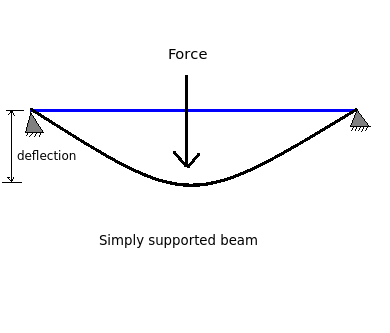# Relation between slope and deflection - Simply supported beam

Q.  Which of the following statements is/are true for a simply supported beam?
- Published on 21 Sep 15

a. Deflection at supports in a simply supported beam is maximum
b. Deflection is maximum at a point where slope is zero
c. Slope is minimum at supports in a simply supported beam
d. All of the above

ANSWER: Deflection is maximum at a point where slope is zero

#### Discussion

• Sravanthi   -Posted on 24 Nov 15
Deflection: The vertical distance between the axis of the beam before and after loading at a point is called as deflection.

- For simply supported beams, deflection is zero at supports and is always maximum at the point where slope is zero.

- For simply supported beams, slope is maximum at supports.

Slope: The angle between tangent to elastic curve and original axis of beam is called as slope. It is measured in radians.## ➨ Post your comment / Share knowledgeEnter the code shown above:

(Note: If you cannot read the numbers in the above image, reload the page to generate a new one.)